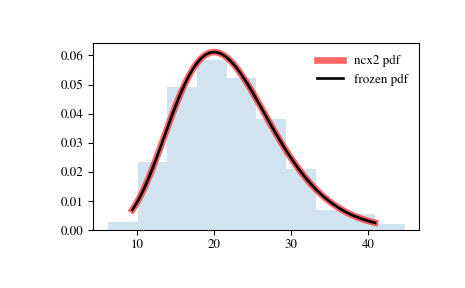# scipy.stats.ncx2¶

scipy.stats.ncx2 = <scipy.stats._continuous_distns.ncx2_gen object>[source]

A non-central chi-squared continuous random variable.

As an instance of the rv_continuous class, ncx2 object inherits from it a collection of generic methods (see below for the full list), and completes them with details specific for this particular distribution.

Notes

The probability density function for ncx2 is:

$f(x, df, nc) = \exp(-\frac{nc+x}{2}) \frac{1}{2} (x/nc)^{(df-2)/4} I[(df-2)/2](\sqrt{nc x})$

for $$x > 0$$.

ncx2 takes df and nc as shape parameters.

The probability density above is defined in the “standardized” form. To shift and/or scale the distribution use the loc and scale parameters. Specifically, ncx2.pdf(x, df, nc, loc, scale) is identically equivalent to ncx2.pdf(y, df, nc) / scale with y = (x - loc) / scale.

Examples

>>> from scipy.stats import ncx2
>>> import matplotlib.pyplot as plt
>>> fig, ax = plt.subplots(1, 1)


Calculate a few first moments:

>>> df, nc = 21, 1.06
>>> mean, var, skew, kurt = ncx2.stats(df, nc, moments='mvsk')


Display the probability density function (pdf):

>>> x = np.linspace(ncx2.ppf(0.01, df, nc),
...                 ncx2.ppf(0.99, df, nc), 100)
>>> ax.plot(x, ncx2.pdf(x, df, nc),
...        'r-', lw=5, alpha=0.6, label='ncx2 pdf')


Alternatively, the distribution object can be called (as a function) to fix the shape, location and scale parameters. This returns a “frozen” RV object holding the given parameters fixed.

Freeze the distribution and display the frozen pdf:

>>> rv = ncx2(df, nc)
>>> ax.plot(x, rv.pdf(x), 'k-', lw=2, label='frozen pdf')


Check accuracy of cdf and ppf:

>>> vals = ncx2.ppf([0.001, 0.5, 0.999], df, nc)
>>> np.allclose([0.001, 0.5, 0.999], ncx2.cdf(vals, df, nc))
True


Generate random numbers:

>>> r = ncx2.rvs(df, nc, size=1000)


And compare the histogram:

>>> ax.hist(r, density=True, histtype='stepfilled', alpha=0.2)
>>> ax.legend(loc='best', frameon=False)
>>> plt.show()Methods

 rvs(df, nc, loc=0, scale=1, size=1, random_state=None) Random variates. pdf(x, df, nc, loc=0, scale=1) Probability density function. logpdf(x, df, nc, loc=0, scale=1) Log of the probability density function. cdf(x, df, nc, loc=0, scale=1) Cumulative distribution function. logcdf(x, df, nc, loc=0, scale=1) Log of the cumulative distribution function. sf(x, df, nc, loc=0, scale=1) Survival function (also defined as 1 - cdf, but sf is sometimes more accurate). logsf(x, df, nc, loc=0, scale=1) Log of the survival function. ppf(q, df, nc, loc=0, scale=1) Percent point function (inverse of cdf — percentiles). isf(q, df, nc, loc=0, scale=1) Inverse survival function (inverse of sf). moment(n, df, nc, loc=0, scale=1) Non-central moment of order n stats(df, nc, loc=0, scale=1, moments=’mv’) Mean(‘m’), variance(‘v’), skew(‘s’), and/or kurtosis(‘k’). entropy(df, nc, loc=0, scale=1) (Differential) entropy of the RV. fit(data, df, nc, loc=0, scale=1) Parameter estimates for generic data. expect(func, args=(df, nc), loc=0, scale=1, lb=None, ub=None, conditional=False, **kwds) Expected value of a function (of one argument) with respect to the distribution. median(df, nc, loc=0, scale=1) Median of the distribution. mean(df, nc, loc=0, scale=1) Mean of the distribution. var(df, nc, loc=0, scale=1) Variance of the distribution. std(df, nc, loc=0, scale=1) Standard deviation of the distribution. interval(alpha, df, nc, loc=0, scale=1) Endpoints of the range that contains alpha percent of the distribution

#### Previous topic

scipy.stats.nakagami

scipy.stats.ncf